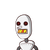# Q8. Find and correct the errors in the following mathematical statement(li) x(3x+2) = 3x² + 2​

Q8. Find and correct the errors in the following mathematical statement

(li) x(3x+2) = 3x² + 2​

### 1 thought on “Q8. Find and correct the errors in the following mathematical statement<br /><br />(li) x(3x+2) = 3x² + 2​”

1.x is to be multiplied.

Step-by-step explanation:

x(3x+2)=3x^2+2

3x^2+2x is not equal to 3x^2+2

To make L.H.S=R.H.S,

We need to multiply x to 2 on the R. H. S

3x^2+2x=3x^2+2×x

Then Equation is true.